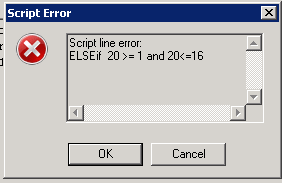# QlikView Scripting

Discussion Board for collaboration on QlikView Scripting.

Announcements

#### Breathe easy -- you now have more time to plan your next steps with Qlik! QlikView 11.2 Extended Support is now valid through December 31, 2020. Click here for more information.Not applicable

## Script line Error : if

Hi :

Can someone help me know what is wrong in my report?

Please find the attached error.

Script:

LET todaytime =  Date(Now(), 'dd');

LET Midstart = NUM(17,'00') ;

LET MidEnd = NUM(31,'00') ;

LET start = NUM(1,'00') ;

LET End = NUM(16,'00') ;

if  \$(vToday) >= \$(Midstart ) and \$(vToday) <=\$(MidEnd )else if \$(vToday) >= \$(start ) and \$(vToday) <=\$(End )thanks

kyoko

1 Solution

Accepted SolutionsMVP

## Re: Script line Error : if

2 small example

LET vToday =  day(Now());

IF \$(vToday) >= 17 and \$(vToday)<=31 THEN

trace '17..31';

ELSEIF \$(vToday) >= 1 and \$(vToday) <=16 THEN

trace '1..16';

ENDIF;

and with variables

LET Midstart = 17;

LET MidEnd = 31;

LET Start = 1;

LET End = 16;

LET vToday =  day(Now())-10;

if  \$(vToday) >= \$(Midstart) and \$(vToday) <=\$(MidEnd) THEN

trace '17..31';

ELSEIF \$(vToday) >= \$(Start) and \$(vToday) <=\$(End) THEN

trace '1..16';

ENDIF;

9 RepliesMVP

## Re: Script line Error : if

Hi,

go to Help Menu and see IF

Regards

Great dreamer's dreams never fulfilled, they are always transcended.Not applicable

## Re: Script line Error : if

HI @max dreamer

How can I go to "Help Menu" in this Community?

thanksMVP

## Re: Script line Error : if

Use ' ' when you call variable..

' \$(vToday)'

Valued Contributor II

## Re: Script line Error : if

Hi Kyoko,

could you please tell me what you have define in vToday variable..

thanks

Valued Contributor II

## Re: Script line Error : if

it seems you have not define variable vToday thats why giving such errorNot applicable

## Re: Script line Error : if

HI AbhaySingh

LET ThisExecTimeDate =  Date(Now(), 'dd');

LET vToday = Num(\$(ThisExecTimeDate), '00');Not applicable

## Re: Script line Error : if

HI @MRKachhiaIMP :

when I use ' \$(vToday)' .MVP

## Re: Script line Error : if

2 small example

LET vToday =  day(Now());

IF \$(vToday) >= 17 and \$(vToday)<=31 THEN

trace '17..31';

ELSEIF \$(vToday) >= 1 and \$(vToday) <=16 THEN

trace '1..16';

ENDIF;

and with variables

LET Midstart = 17;

LET MidEnd = 31;

LET Start = 1;

LET End = 16;

LET vToday =  day(Now())-10;

if  \$(vToday) >= \$(Midstart) and \$(vToday) <=\$(MidEnd) THEN

trace '17..31';

ELSEIF \$(vToday) >= \$(Start) and \$(vToday) <=\$(End) THEN

trace '1..16';

ENDIF;Not applicable

## Re: Script line Error : if

If your working with variables & varibales holds number use \$(vName) or vName. If the variable holds string use '\$(vName)'